# Descriptive Statistic

• Last Updated : 22 Apr, 2020

In Descriptive statistics, we are describing our data with the help of various representative methods like by using charts, graphs, tables, excel files etc. In descriptive statistics, we describe our data in some manner and present it in a meaningful way so that it can be easily understood. Most of the times it is performed on small data sets and this analysis helps us a lot to predict some future trends based on the current findings. Some measures that are used to describe a data set are measures of central tendency and measures of variability or dispersion.

Types of Descriptive statistic:

• Measure of central tendency
• Measure of variability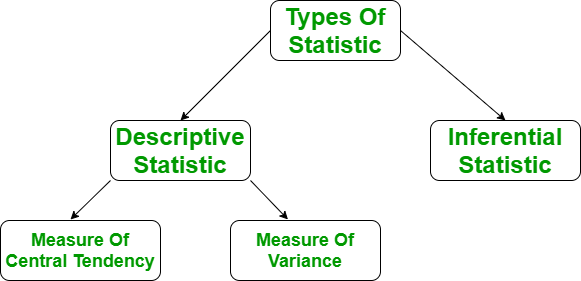Measure of central tendency:
It represents the whole set of data by single value.It gives us the location of central points. There are three main measures of central tendency:

• Mean
• Mode
• Median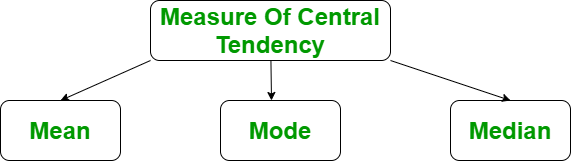1. Mean:

It is the sum of observation divided by the total number of observations. It is also defined as average which is the sum divided by count.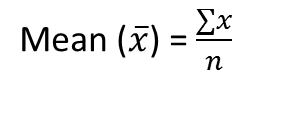where, n = number of terms
Python Code to find Mean in python

 `import` `numpy as np`` ` `# Sample Data``arr ``=` `[``5``, ``6``, ``11``]      ``# Mean``mean ``=` `np.mean(arr)      `` ` `print``(``"Mean = "``, mean)`

Output :

```Mean =  7.333333333333333
```
2. Mode:
It is the value that has the highest frequency in the given data set. The data set may have no mode if the frequency of all data points is the same. Also, we can have more than one mode if we encounter two or more data points having the same frequency.

Code to find Mode in python

 `from` `scipy ``import` `stats`` ` `# sample Data``arr ``=``[``1``, ``2``, ``2``, ``3``]     `` ` `# Mode``mode ``=` `stats.mode(arr)      ``print``(``"Mode = "``, mode)`

Output:

`Mode =  ModeResult(mode=array(), count=array())`
3. Median:
It is the middle value of the data set. It splits the data into two halves. If the number of elements in the data set is odd then the centre element is median and if it is even then the median would be the average of two central elements.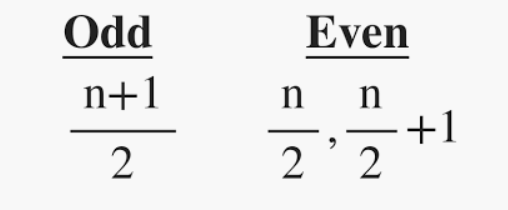where, n=number of terms
Python code to find Median

 `import` `numpy as np`` ` `# sample Data``arr ``=``[``1``, ``2``, ``3``, ``4``]    `` ` `# Median``median ``=` `np.median(arr)   `` ` `print``(``"Median = "``, median)`

Output:

```Median =  2.5
```
4. Measure of variability:
Measure of variability is known as the spread of data or how well is our data is distributed. The most common variability measures are:

• Range
• Variance
• Standard deviation

•1. Range:

The range describes the difference between the largest and smallest data point in our data set. The bigger the range, the more is the spread of data and vice versa.

Range = Largest data value – smallest data value

Python Code to find Range

 `import` `numpy as np`` ` `# Sample Data``arr ``=` `[``1``, ``2``, ``3``, ``4``, ``5``]     `` ` `#Finding Max``Maximum ``=` `max``(arr)          ``# Finding Min ``Minimum ``=` `min``(arr) `` ` `# Difference Of Max and Min          ``Range` `=` `Maximum``-``Minimum     ``print``(``"Maximum = {}, Minimum = {} and Range = {}"``.``format``(``        ``Maximum, Minimum, ``Range``))`

Output:

`Maximum = 5, Minimum = 1 and Range = 4`
2. Variance:
It is defined as an average squared deviation from the mean. It is being calculated by finding the difference between every data point and the average which is also known as the mean, squaring them, adding all of them and then dividing by the number of data points present in our data set.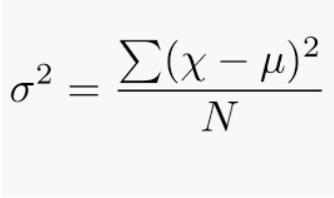where N = number of terms
u = Mean
Python code to find Variance

 `import` `statistics `` ` `# sample data ``arr ``=` `[``1``, ``2``, ``3``, ``4``, ``5``]     ``# variance``print``(``"Var = "``, (statistics.variance(arr)))     `

Output:

`Var =  2.5`
3. Standard Deviation:
It is defined as the square root of the variance. It is being calculated by finding the Mean, then subtract each number from the Mean which is also known as average and square the result. Adding all the values and then divide by the no of terms followed the square root.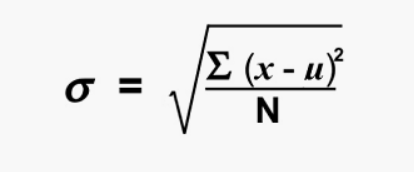where N = number of terms
u = Mean
Python code to perform Standard Deviation:

 `import` `statistics `` ` `# sample data ``arr ``=` `[``1``, ``2``, ``3``, ``4``, ``5``]     ``# Standard Deviation``print``(``"Std = "``, (statistics.stdev(arr)))    `

Output:

`Std = 1.5811388300841898`

References :
Big Data Wikipedia
Formulae

My Personal Notes arrow_drop_up